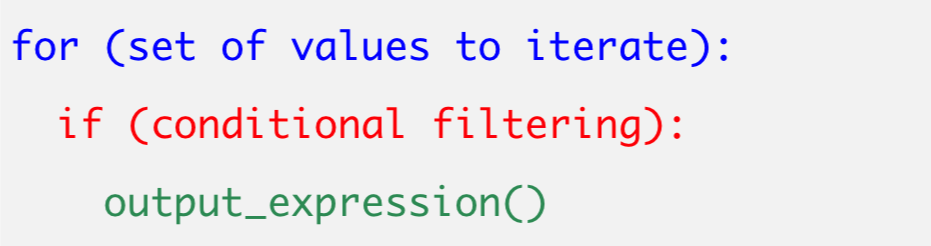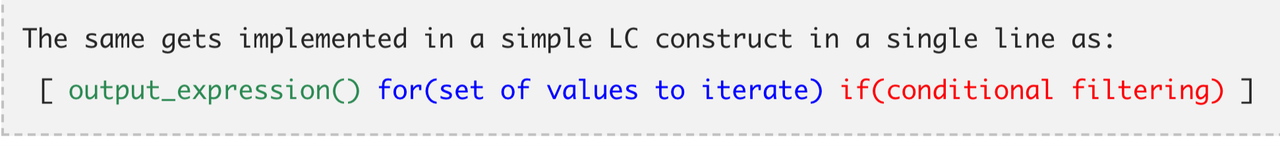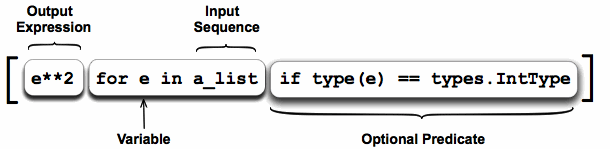# 10 Python List Comprehension Practice Exercises That Will Make You A Hiring Manager's Dream# How can a python newbie can stand out in a sea of competition? Show your list comprehension

As a Software Quality Assurance Engineer, I use python as one tool in my test automation tool chest. List comprehension is one of the python techniques that not only adds flair to your code, it also saves cpu cycles and is considered ‘Pythonic’.

## What is list comprehension?

The list comprehension syntax is somewhat of a compressed ‘for’ loop.

### Classic ‘for’ loop

Given a list of numbers, remove all odd numbers from the list:

``````numbers = [3,5,45,97,32,22,10,19,39,43]
result = []
for number in numbers:
if number % 2 == 0:
result.append(number)
print(result)
``````

### The list comprehension way

``````result = [number for number in numbers if number % 2 == 0]
print(result)
``````

So glorious, so pretty, so pythonic ;)

## List comprehension syntax

The trickiest part of learning list comprehension for me was really understanding the syntax. The best method of explaining IMO is from Tutorial – Python List Comprehension With Examples

A traditional for loop:Translated to list comprehension:And another great visual## Examples

### Given a list of numbers, remove floats (numbers with decimals)

``````original_list = [2,3.75,.04,59.354,6,7.7777,8,9]
only_ints = [number for number in original_list if type(number) == int]
print(only_ints)
``````

## Practice

I needed practice, so I used this list. Each challenge below links to a solution gist.

# Contact Me

Written on December 26, 2018